## RS Aggarwal Class 8 Solutions Chapter 11 Compound Interest Ex 11D

These Solutions are part of RS Aggarwal Solutions Class 8. Here we have given RS Aggarwal Solutions Class 8 Chapter 11 Compound Interest Ex 11D.

Other Exercises

Tick the correct answer in each of the following

Question 1.
Solution:
Principal (P) = Rs. 5000
Rate (R) = 8% p.a.
Period (n) = 2 yearsQuestion 2.
Solution:
Principal (P) = Rs. 10000
Rate (R) = 10% p.a.
Period (n) = 3 yearsQuestion 3.
Solution:
Principal (P) = Rs. 10000
Rate (R) = 12% p.a.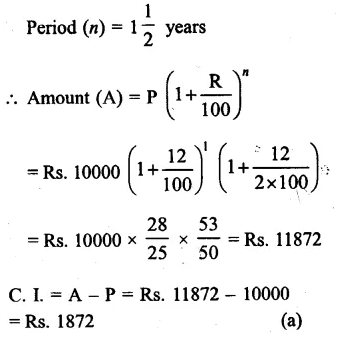Question 4.
Solution:
Principal (P) = Rs. 4000
Rate (R) = 10% p.a.
Period (a) = 2 years 3 monthsQuestion 5.
Solution:
Principal (P) = Rs. 25000
Rate (R1) = 5% for the first year
R2 = 6% for the second year
R3 = 8% for the third yearQuestion 6.
Solution:
Principal (P) = Rs. 6250
Rate (R) = 8% p.a. or 4% half yearly
Period (n) = 1 year or 2 half yearsQuestion 7.
Solution:
Principal (P) = Rs. 40000
Rate (R) = 6% p.a. $$\\ \frac { 6 }{ 4 }$$ = $$\\ \frac { 3 }{ 2 }$$ % quarterly
Period (n) = 6 months = 2 quarters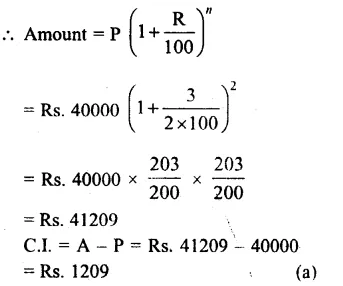Question 8.
Solution:
Present population (P) = 24000
Rate of increase (R) = 5% p.a.
Period (n) = 2 years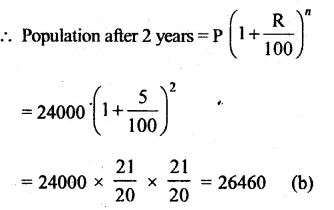Question 9.
Solution:
3 years ago, the value of machine = Rs. 60000
Rate of depreciation (R) = 10%
Period (n) = 3 yearsQuestion 10.
Solution:
Present value = Rs. 40000
Rate of depreciation (R) = 20% p.a.
Value of machine 2 years ago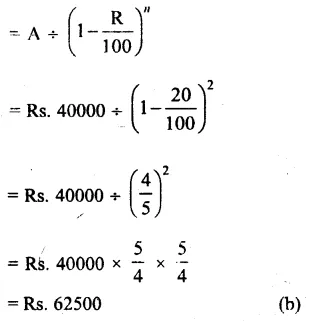Question 11.
Solution:
Rate of growth in population (R) = 10%
Present population = 33275
Population 3 years ago = A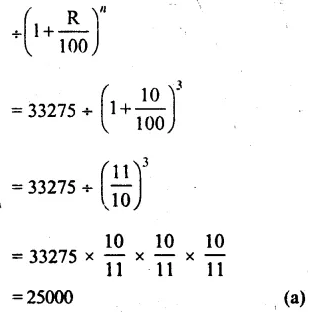Question 12.
Solution:
S.I. = Rs. 1200
Rate (R) = 5%
Period (T) = 3 yearsQuestion 13.
Solution:
C.I. on a sum = Rs. 510
Rate (R) = $$12\frac { 1 }{ 2 }$$ % = $$\\ \frac { 25 }{ 2 }$$ % p.a.
Period (n) = 2 years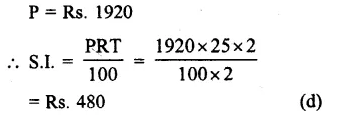Question 14.
Solution:
Amount = Rs. 4913
Rate (R) = $$6\frac { 1 }{ 4 }$$ = $$\\ \frac { 25 }{ 4 }$$ %
Period (n) = 3 yearsQuestion 15.
Solution:
Sum (P) = Rs. 7500
Amount (A) = 8427
Period = 2 years
Let R be the rate of p.a., then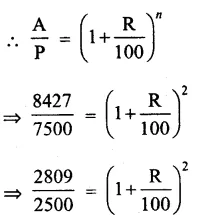Hope given RS Aggarwal Solutions Class 8 Chapter 11 Compound Interest Ex 11D are helpful to complete your math homework.

If you have any doubts, please comment below. Learn Insta try to provide online math tutoring for you.